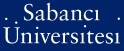# Spin accumulation in diffusive conductors with Rashba and Dresselhaus spin-orbit interaction

Duckheim, Mathias and Loss, Daniel and Scheid, Matthias and Richter, Klaus and Adagideli, İnanç and Jacquod, Philippe (2010) Spin accumulation in diffusive conductors with Rashba and Dresselhaus spin-orbit interaction. Physical Review B: Condensed Matter and Materials Physics, 81 (8). ISSN 1098-0121Preview
PDF (This is a RoMEO green publisher -- author can archive post-print (ie final draft post-refereeing) and publisher's version/PDF) - Requires a PDF viewer such as GSview, Xpdf or Adobe Acrobat Reader
271Kb

Official URL: http://dx.doi.org/10.1103/PhysRevB.81.085303

## Abstract

We calculate the electrically induced spin accumulation in diffusive systems due to both Rashba (with strength $\alpha)$ and Dresselhaus (with strength $\beta)$ spin-orbit interaction. Using a diffusion equation approach we find that magnetoelectric effects disappear and that there is thus no spin accumulation when both interactions have the same strength, $\alpha=\pm \beta$. In thermodynamically large systems, the finite spin accumulation predicted by Chaplik, Entin and Magarill, [Physica E {\bf 13}, 744 (2002)] and by Trushin and Schliemann [Phys. Rev. B {\bf 75}, 155323 (2007)] is recovered an infinitesimally small distance away from the singular point $\alpha=\pm \beta$. We show however that the singularity is broadened and that the suppression of spin accumulation becomes physically relevant (i) in finite-sized systems of size $L$, (ii) in the presence of a cubic Dresselhaus interaction of strength $\gamma$, or (iii) for finite frequency measurements. We obtain the parametric range over which the magnetoelectric effect is suppressed in these three instances as (i) $|\alpha|-|\beta| \lesssim 1/mL$, (ii)$|\alpha|-|\beta| \lesssim \gamma p_{\rm F}^2$, and (iii) $|\alpha|-|\beta| \lesssiM \sqrt{\omega/m p_{\rm F}\ell}$ with $\ell$ the elastic mean free path and $p_{\rm F}$ the Fermi momentum. We attribute the absence of spin accumulation close to $\alpha=\pm \beta$ to the underlying U (1) symmetry. We illustrate and confirm our predictions numerically.

Item Type: Article Q Science > Q Science (General)Q Science > QC Physics > QC501-766 Electricity and magnetism Q Science > QC Physics > QC176-176.9 Solids. Solid state physics Q Science > QC Physics 13761 İnanç Adagideli 16 Feb 2010 11:20 24 Jul 2019 15:42

### Available Versions of this Item

Repository Staff Only: item control page